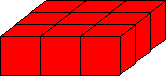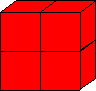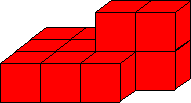Problem Statement

You are given 2 rectangular parallelepipeds (rectangular solids) in space, edges parallel to coordinate axes. Compute the volume of their union.

For example, consider the two following parallelepipeds (they are divided into unit cubes for clarity):andWhen combined in the following manner, their union is a figure of volume 12 (it consists of 12 unit blocks: ten on the bottom level, and two on the top level):You are given a String[] parallelepipeds containing exactly two elements. Each element represents a single parallelepiped, and is formatted as "x1 y1 z1 x2 y2 z2" (quotes for clarity only), where (x1, y1, z1) and (x2, y2, z2) are the coordinates of opposing corners of the parallelepiped.

Definition

 Class: ParallelepipedUnion Method: getVolume Parameters: String[] Returns: int Method signature: int getVolume(String[] parallelepipeds) (be sure your method is public)

Constraints

-parallelepipeds will contain exactly 2 elements.
-Each element of parallelepipeds will be formatted as "x1 y1 z1 x2 y2 z2" (quotes for clarity only).
-In each element of parallelepipeds, x1 < x2, y1 < y2, z1 < z2.
-All coordinates will be integers between 1 and 100, inclusive, without extra leading zeroes.

Examples

0)

 {"1 1 1 4 4 2", "3 2 1 5 3 3"}
Returns: 12
 The example from the problem statement.
1)

 {"1 1 1 2 2 2", "3 3 3 4 4 4"}
Returns: 2
 Two unit cubes that do not intersect.
2)

 {"1 10 20 5 15 23", "2 12 22 3 14 23"}
Returns: 60
 One parallelepiped inside another.
3)

 {"28 31 21 67 60 81","60 1 21 67 50 81"}
Returns: 80460
4)

 {"34 3 50 37 6 53","36 1 51 38 4 55"}
Returns: 49

Problem url:

http://www.topcoder.com/stat?c=problem_statement&pm=6498

Problem stats url:

http://www.topcoder.com/tc?module=ProblemDetail&rd=9992&pm=6498

Petr

Testers:

PabloGilberto , brett1479 , Yarin , Olexiy

Problem categories:

Geometry, Simple Math